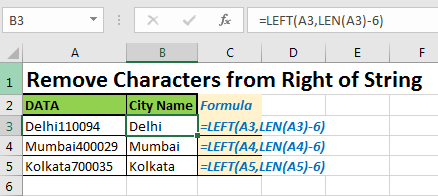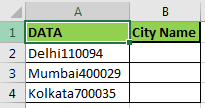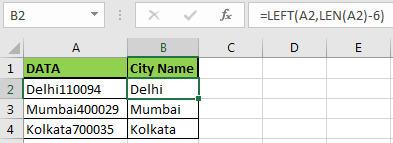# How to Remove Characters From Right in Excel

So, many times we get data that has some additional data attached to the right that we don’t actually require. So we get a need to get rid of that extra text. In this article we learn how to remove characters from the right of the text.Generic Formula to Remove Characters from Right

=LEFT(text,LEN(text)-num_of_char)

Text: the text from which you want to remove the last n characters in excel
Num_of_char: the num of chars you want to remove.

Basically what we are doing is basic mathematics. Instead of removing n characters from right we show total_characters-n_characters_from_right from left. Which gives the same result. Let's see an example to understand it better.

#### Excel Remove Characters From Right Example

So in this example, we are removing city codes that are attached with city names here.We know that the city code is of 6 characters. To extract the city name we need to remove 6 characters from the string we will use above the below formula.

Write this formula in cell B2 and copy in below cells.

=LEFT(A2,LEN(A2)-6)#### How does it work?

This is easy. We know how the LEFT function in excel works.
LEFT(string,num_char) extracts the given number of characters from the left of a given string. So we give it the string first.

Now we need to calculate the number of characters to be extracted. To do so we use LEN(string) function that returns the total number of characters in a string.t

Then we subtract 6 from the total number of characters, which means that 6 characters are removed from the right of the string. In the end, we get our city name.

#### Now some frequently asked questions?

How to remove the last character from string in excel?
To remove the last character from string using below formula:

=LEFT(A2,LEN(A2)-1)

Similarly, to remove two-character replace 1 by 2 and so on.

So yeah guys, this is the easiest way to remove characters from the right of a text. Let me know if this was helpful. If it wasn’t, then definitely let me know in the comment section below. I’ll be happy to solve the problem.

Related Articles:

How to Remove leading and trailing spaces from the text in Excel

How to use the RIGHT function in Excel

How to Remove unwanted characters in Excel

How to Extract Text From A String In Excel Using Excel’s LEFT And RIGHT Function in Excel

Popular Articles:

50 Excel Shortcuts to Increase Your Productivity

How to use the VLOOKUP Function in Excel

How to use the COUNTIF function in Excel

How to use the SUMIF Function in Excel

Terms and Conditions of use

The applications/code on this site are distributed as is and without warranties or liability. In no event shall the owner of the copyrights, or the authors of the applications/code be liable for any loss of profit, any problems or any damage resulting from the use or evaluation of the applications/code.